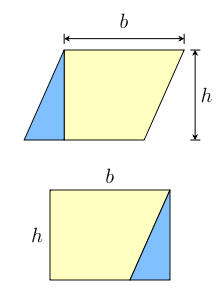# C Program Area Of Parallelogram | C Programs

Another program in the list of C Programs – C program to find the area of a parallelogram, with sample outputs and sample example code. Do check it out.

In this appropriate article, we will learn the numerous methods to calculate the area of a parallelogram in C programming. The ways to calculate the area of a parallelogram in C Programming are as follows:

• Using Standard Method
• Using Function
• Using Pointers
• Using Macros

As we all know, a parallelogram is a 2-dimensional closed figure made up of four sides. There is a multitude of characteristics of a parallelogram.

A parallelogram is a quadrilateral whose opposite sides are equal and parallel to each other. The opposite angles of a parallelogram are equal in nature.

The sum of all the angles of a parallelogram is equal to 360 degrees.

A parallelogram looks like this:The formula to calculate the area of a parallelogram = Base * Height

The height of a parallelogram is equal to the minimum distance between the opposite sides.

The various methods used to calculate the area of a parallelogram in C programming are as follows:

## Using Standard Method

1)By calculating the area of parallelogram we need a base and height.

2)We declared the variables base, height, area.

3)Substitute the values into the formula area=(base*height), then we will get area value, that will be assigned to the variable “area”.

output:

### Using Function

1)We are calling the function as area(b,h), here we are passing the b,h values as arguments.

2)The called function area(float b, float h) will calculate the area and returns the value.

3)The return value will be assigned to the variable “a”.

output:

#### Using Pointers

1)We are calling the function by passing references as arguments using area(&b,&h,&a).

2)The called function will calculate the area, which is having pointers as arguments.

3)The calculated area will be assigned to the pointer variable “area’.

output:

##### Using Macros

1)Here area(b,h) is the symbolic name to the expression (b*h).

2)area(b,h)replaced with that expression given at #define.

output:
x

## C Program To Print Number Of Days In A Month | Java Tutoring

C program to input the month number and print the number of days in that ...